Mechanics

# Traction

Traction or tension is the name given to forces applied through wires, cables or ropes . Traction is a contact force that is transmitted through a physical medium capable of pulling or pulling distant bodies. It enables the transfer of forces between different bodies, but also allows the direction and direction of transmitted forces to be configured as needed. For this reason, there are numerous problems and exercises that involve the application of tensile forces, such as in block systems, inclined planes , pulleys , etc.

Because it is a force, the unit of measure of traction, according to the International System of Units , is the newton (N) Furthermore, the pull that is exerted on a system of bodies can be calculated by applying Newton’s three laws.

## What is traction?

Tension is a force – therefore, a vector quantity – that can be transmitted by ropes, wires and cables. When tension is applied to a body, it is pulled in the same direction as the cable. To calculate the magnitude of the tensile force, it is necessary to apply Newton’s second law to each of the spatial directions that are relevant to the problem to be solved.

In order to understand the concept of traction, let’s deal with the ideal situation, as simple as possible: a body of mass m pulled by an inextensible cord (which cannot be stretched) and of negligible mass, which is supported on a flat, horizontal and free-standing surface. friction :

In the figure above, we see that there are three forces acting on the block: normal (N), weight (P) and traction (T). Since the resultant of the forces in the vertical direction is zero, the normal and weight forces cancel each other out, so that the magnitude of the resultant force is equal to the magnitude of the tension T itself. In this way, according to Newton’s 2nd law , the tension can be equated to the mass of the block multiplied by the acceleration. Watch: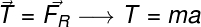R – resultant force (N)

m – mass (kg)

a – acceleration (m/s²)

## Traction and friction force

Let’s analyze the situation in which a block of mass m, placed on a frictionless horizontal surface, is pulled by a traction parallel to the horizontal plane, as shown in the figure:

In the case illustrated above, we have three possibilities:

• Block at rest;
• Block in uniform rectilinear motion (constant speed);
• Block in accelerated motion.

In the first and second cases, the resultant force must be equal to zero, since the acceleration of the block is zero, however, the friction force is different for each of these cases, since the first case involves a situation of static friction, and the second, dynamic or kinetic friction .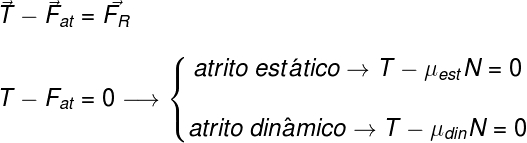at – friction force (N)

μ EST – coefficient of static friction

μ DIN – dynamic friction coefficient

N – normal force (N)

In the third case, where the block moves with non-zero acceleration, the friction is kinetic or dynamic and the resultant force is non-zero, so: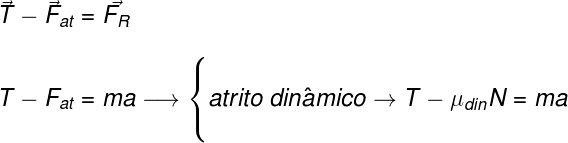## Systems of bodies pulled by a horizontal traction

It is possible that you are trying to solve some system of bodies pulled by an external force. If this is the case, don’t worry: these cases are quite simple to solve. First, since all the bodies move together, you can imagine them as a single body, to do this, circle them and observe what external forces act on the system. See the figure below, in which we show a system with two bodies connected by an inextensible string. One of these bodies is being pulled by an external pulling force:

1.2 – traction that block 1 makes on block 2

2.1 – traction that block 2 makes on block 1

The figure shows two blocks, m 1 in 2 , connected by a cable. Block m 2 is pulled by an external force T. Since these blocks are always at the same distance, we can consider them as a single rigid body of mass m = (m 1 + m 2 ). For this, we need to remember that the traction that block 1 exerts on block 2 (T 1.2 ) is equal, but contrary to the traction T 2.1 and that, according to Newton’s third law , the law of action and reaction , must be cancelled. Knowing this, the traction T acting on the m 1 in 2 block system is equal to: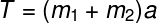If we want to know what the resultant forces are on each of the blocks, we must apply Newton’s second law to each of them. We will get the following result: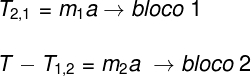## Traction on the inclined plane

When a body is supported on an inclined plane and is subjected to a traction force, it is necessary to decompose the forces acting on it . In this case, it is necessary to adopt a reference system whose horizontal direction coincides with the direction of the plane, so that the vertical direction coincides with the direction of the normal force acting on the block. Doing this, we will notice that the force pulling the block is opposite to the x component of the weight force (P x ) of that block.

it is also possible to notice that the y component of the weight (P y ) is the side adjacent to the angle θ, therefore it is equal to the magnitude of the weight force multiplied by the cosine of this angle. Furthermore, it is noted that the normal force vanishes with the P y component. . Here’s how the resultant force is calculated in the x and y directions: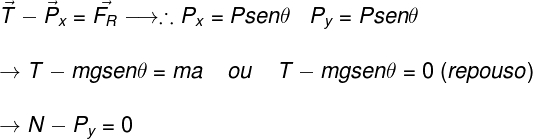On the inclined plane, we need to find the resultant force in the x and y directions.

## Simple pendulum pull

The simple pendulum is a movement that occurs when a body is made to oscillate under the action of the force of weight and a traction. The resultant force on the oscillating body is never zero, since it describes a circular motion and, therefore, presents a centripetal force . This force, in turn, always points to the center of the trajectory and is equal to the resultant force on the body. The figure below shows a body performing a pendular motion. It is possible to observe that the traction has its maximum value when the body is in the lowest position of the trajectory. Watch:

## Solved exercises on traction

Question 1 – (PUC-MG) An elevator manufacturer establishes, for safety reasons, that the force applied to the steel cables that support its elevators cannot exceed 1.2.10 4 N. Consider one of these elevators with a total mass of 1.0 x 10 4 kg (mass of the elevator with passengers) and assume g = 10 m/s². Under these conditions, the maximum acceleration of the elevator on the way up cannot exceed:

a) 1.2 m/s²

b) 2.0 m/s²

c) 5.0 m/s²

d) 9.8 m/s²

Template: letter b.

Resolution:

The exercise describes a situation in which the pulling force and the weight force act in the same direction, but in opposite directions. Thus, the resolution of this exercise is relatively simple: just notice that the difference between the traction force and the weight force is equal to the resultant force and, therefore, is equal to the product of the mass and the acceleration. Furthermore, to find out what is the maximum acceleration at which the elevator can go up, we simply substitute the maximum traction module, informed by the exercise. Watch: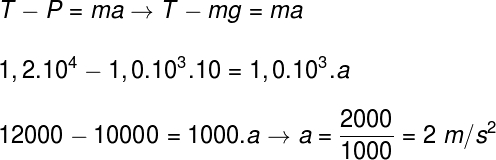Based on the calculation performed, we find that the maximum acceleration with which the elevator can rise is equal to 2 m/s².

Question 2 – (UFRGS) Two blocks, of masses m 1 = 3.0 kg in 2 = 1.0 kg, connected by an inextensible string, can slide without friction on a horizontal plane. These blocks are pulled by a horizontal force F of magnitude F = 6 N, as shown in the following figure.

The resulting forces on m 1 in 2 are, respectively:

a) 3.0 N and 1.5 N

b) 4.5 N and 1.5 N

c) 4.5 N and 3.0 N

d) 6.0 N and 3.0 N

e) 6.0 N and 4.5 N

Template: letter B.

Resolution:

To solve the exercise, we first consider the block system as a single rigid body. After that, we must calculate what is the acceleration at which this system moves: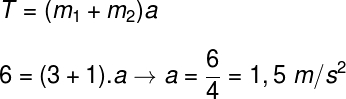Then it is necessary to calculate what is the resultant force in each of the blocks. To do so, notice that, on the block on the left, the only force acting in the horizontal direction is the pull exerted by the block on the right, so this force is the resultant. Watch: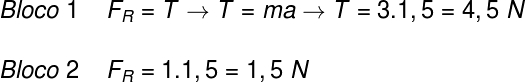The calculations performed indicate that the resulting forces in each of the blocks correspond to 4.5 N and 1.5 N.